# Term 1 Sample Paper Physics for Class 12 2021-22

### Term 1 Sample Paper Physics for Class 12 2021-22

Sample Question Paper 2021-22
Term 1
Subject: Physics (042)
Time: 90 Minutes Max. Marks 35
General Instructions:
1. The Question Paper contains three sections.
2. Section A has 25 questions. Attempt any 20 questions.
3. Section B has 24 questions. Attempt any 20 questions.
4. Section C has 6 questions. Attempt any 5 questions.
5. All questions carry equal marks.
6. There is no negative marking.

SECTION A

This section consists of 25 multiple choice questions with overall choice to attempt any 20 questions. In case more than desirable numberof questions are attempted, ONLY first 20 will be considered for evaluation.

Q 1. Which of the following is NOT the property of equipotential surface?

i) They do not cross each other.

ii) The rate of change of potential with distance on them is zero.

iii) For a uniform electric field they are concentric spheres.

iv) They can be imaginary spheres.

iii) For a uniform electric field they are concentric spheres.

Q 2. Two point charges +8q and -2q are located at x=0 and x=L respectively. The point on x axis at which net electric field is zero due to these charges is-

i) 8L

ii) 4L

iii) 2 L

iv) L

iii) 2 L

Q 3. An electric dipole of moment p is placed parallel to the uniform electric field. The amount of work done in rotating the dipole by 900 is-

i) 2pE

ii) pE

iii) pE/2

iv) Zero

ii) pE

Q 4. Three capacitors 2µF, 3µF and 6µF are joined in series with each other. The equivalent capacitance is-

i) 1/2µF

ii) 1µF

iii) 2µF

iv) 11µF

ii) 1µF

Q 5. Two point charges placed in a medium of dielectric constant 5 are at a distance r between them, experience an electrostatic force ‘F’. The electrostatic force between them in vacuum at the same distance r will be-

i) 5F

ii) F

iii) F/2

iv) F/5

i) 5F

Q 6. Which statement is true for Gauss law-

i) All the charges whether inside or outside the gaussian surface contribute to the electric flux.

ii) Electric flux depends upon the geometry of the gaussian surface.

iii) Gauss theorem can be applied to non-uniform electric field.

iv) The electric field over the gaussian surface remains continuous and uniform at every point.

iv) The electric field over the gaussian surface remains continuous and uniform at every point.

Q 7. A capacitor plates are charged by a battery with ‘V’ volts. After charging battery is disconnected and a dielectric slab with dielectric constant ‘K’ is inserted between its plates, the potential across the plates of a capacitor will become-

i) Zero

ii) V/2

iii) V/K

iv) KV

iii) V/K

Q 8. The best instrument for accurate measurement of EMF of a cell is-

i) Potentiometer

ii) metre bridge

iii) Voltmeter

iv) ammeter and voltmeter

i) Potentiometer

Q 9. An electric current is passed through a circuit containing two wires of same material, connected in parallel. If the lengths and radii of the wires are in the ratio of 3:2 and 2:3, then the ratio of the current passing through the wire will be-

i) 2:3

ii) 3:2

iii) 8:27

iv) 27:8

iii) 8:27

Q 10. By increasing the temperature, the specific resistance of a conductor and a semiconductor-

i) increases for both

ii) decreases for both

iii) increases for a conductor and decreases for a semiconductor

iv) decreases for a conductor and increases for a semiconductor.

iii) increases for a conductor and decreases for a semiconductor

Q 11. We use alloys for making standard resistors because they have

i) low temperature coefficient of resistivity and high specific resistance

ii) high temperature coefficient of resistivity and low specific resistance

iii) low temperature coefficient of resistivity and low specific resistance

iv) high temperature coefficient of resistivity and high specific resistance

i) low temperature coefficient of resistivity and high specific resistance

Q 12. A constant voltage is applied between the two ends of a uniform metallic wire, heat ‘H’ is developed in it. If another wire of the same material, double the radius and twice the length as compared to original wire is used then the heat developed in it will be-

i) H/2

ii) H

iii) 2H

iv) 4H

iii) 2H

Q 13. If the potential difference V applied across a conductor is increased to 2V with its temperature kept constant, free electrons in a conductor

i) remain the same

ii) become half of its previous value

iii) be double of its initial value

iv) become zero.

ii) become half of its previous value

Q 14. The equivalent resistance between A and B is-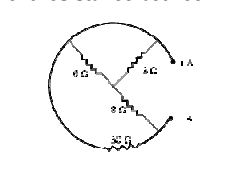i) 3 ohms

ii) 5.5 ohms

iii) 7.5 ohms

iv) 9.5 ohms

iii) 7.5 ohms

Q 15. The SI unit of magnetic field intensity is

i) AmN-1

ii) NA-1m -1

iii) NA-2m -2

iv) NA-1m -2

ii) NA-1m -1

Q 16. The coil of a moving coil galvanometer is wound over a metal frame in order to

i) reduce hysteresis

ii) increase sensitivity

iii) increase moment of inertia

iv) provide electromagnetic damping

iv) provide electromagnetic damping

Q 17. Two wires of the same length are shaped into a square of side ‘a’ and a circle with radius ‘r’. If they carry same current, the ratio of their magnetic moment is-

i) 2 : π

ii) π : 2

iii) π : 4

iv) 4 : π

iii) π : 4

Q 18. The horizontal component of earth’s magnetic field at a place is √3 times the vertical component. The angle of dip at that place is-

i) π/6

ii) π/3

iii) π/4

iv) 0

i) π/6

Q 19. The small angle between magnetic axis and geographic axis at a place is-

i) Magnetic meridian

ii) Geographic meridian

iii) Magnetic inclination

iv) Magnetic Declination

iv) Magnetic Declination

Q 20. Two coils are placed close to each other. The mutual inductance of the pair of coils depends upon the

i) rate at which current change in the two coils

ii) relative position and orientation of the coils

iii) rate at which voltage induced across two coils

iv) currents in the two coils

ii) relative position and orientation of the coils

Q 21. A conducting square loop of side ‘L’ and resistance ‘R’ moves in its plane with the uniform velocity ‘v’ perpendicular to one of its sides. A magnetic induction ‘B’ constant in time and space pointing perpendicular and into the plane of the loop exists everywhere as shown in the figure. The current induced in the loop is-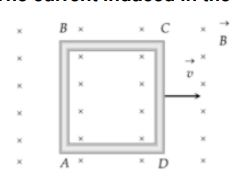i) BLv/R Clockwise

ii) BLv/R Anticlockwise

iii) 2BLv/R Anticlockwise

iv)Zero

iv)Zero

Q 22. The magnetic flux linked with the coil (in Weber) is given by the equation –Փ = 5t2+ 3t + 16

The induced EMF in the coil at time, t=4 will be-

i) -27 V

ii) -43 V

iii) -108 V

iv) 210 V

ii) -43 V

Q 23. Which of the following graphs represent the variation of current(I) with frequency (f) in an AC circuit containing a pure capacitor?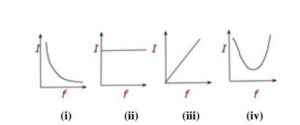(iii)

Q 24. A 20 volt AC is applied to a circuit consisting of a resistance and a coil with negligible resistance. If the voltage across the resistance is 12 volt, the voltage across the coil is-

i) 16 V

ii) 10 V

iii) 8 V

iv) 6 V

i) 16 V

Q 25. The instantaneous values of emf and the current in a series ac circuit are-

E = Eo Sin ωt and I= Io sin( ωt+π/3) respectively, then it is

i) Necessarily a RL circuit

ii) Necessarily a RC circuit

iii)Necessarily a LCR circuit

iv) Can be RC or LCR circuit

iv) Can be RC or LCR circuit

SECTION B

This section consists of 24 multiple choice questions with overall choice to attempt any 20 questions. In case more than desirable number of questions are attempted, ONLY first 20 will be considered for evaluation.

Q 26. A cylinder of radius r and length l is placed in an uniform electric field parallel to the axis of the cylinder. The total flux for the surface of the cylinder is given by-

i) zero

ii) π r2

iii) E π r2

iv) )2 Eπ r2

i) zero

Q 27. Two parallel large thin metal sheets have equal surface densities 26.4 x 10-12 C/m2 of opposite signs. The electric field between these sheets is-

i) 1.5N/C

ii) 1.5 x 10-16 N/C

iii) 3 x 10-10N/C

iv) 3N/C

iv) 3N/C

Q 28. Consider an uncharged conducting sphere. A positive point charge is placed outside the sphere. The net charge on the sphere is then,

i) negative and uniformly distributed over the surface of sphere

ii) positive and uniformly distributed over the surface of sphere

iii) negative and appears at a point the surface of sphere closest to point charge

iv) Zero

iv) Zero

Q 29. Three Charges 2q, -q and -q lie at vertices of a triangle. The value of E and V at centroid of triangle will be-

i) E#0 and V#0

ii) E=0 and V=0

iii) E#0 and V=0

iv) E=0 and V#0

iii) E#0 and V=0

Q 30. Two parallel plate capacitors X and Y, have the same area of plates and same separation between plates. X has air and Y with dielectric of constant 2 , between its plates. They are connected in series to a battery of 12 V. The ratio of electrostatic energy stored in X and Y is-

i) 4:1

ii) 1:4

iii) 2:1

iv) 1:2

iii) 2:1

Q 31. Which among the following, is not a cause for power loss in a transformer-

i) Eddy currents are produced in the soft iron core of a transformer.

ii) Electric Flux sharing is not properly done in primary and secondary coils.

iii) Humming sound produed in the tranformers due to magnetostriction.

iv) Primary coil is made up of a very thick copper wire.

iv) Primary coil is made up of a very thick copper wire.

Q 32. An alternating voltage source of variable angular frequency ‘w’ and fixed amplitude ‘V’ is connected in series with a capacitance C and electric bulb of resistance R(inductance zero). When ‘w’ is increased-

i) The bulb glows dimmer.

ii) The bulb glows brighter.

iii) Net impedance of the circuit remains unchanged.

iv) Total impedance of the circuit increases.

ii) The bulb glows brighter.

Q 33. A solid spherical conductor has charge +Q and radius R. It is surrounded by a solid spherical shell with charge -Q, innerradius 2R, and outer radius 3R. Which of the following statements is true?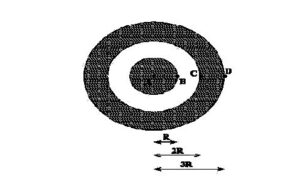i)The electric potential has a maximum magnitude at C and the electric field has a maximum magnitude at A

ii) The electric potential has a maximum magnitude at D and the electric field has a maximum magnitude at B.

iii) The electric potential at A is zero and the electric field has a maximum magnitude at D.

iv) Both the electric potential and electric field achieve a maximum magnitude at B.

iv) Both the electric potential and electric field achieve a maximum magnitude at B.

Q 34. A battery is connected to the conductor of non-uniform cross section area. The quantities or quantity which remains constant is-

i) electric field only

ii) drift speed and electric field

iii)electric field and current

iv) current only

iv) current only

Q 35. Three resistors having values R1, R2, and R3 are connected in series to a battery. Suppose R1 carries a current of 2.0 A, R2 has a resistance of 3.0 ohms, and R3 dissipates 6.0 watts of power. Then the voltage across R3 is-

i) 1V

ii) 2V

iii) 3V

iv) 4V

iii) 3V

Q 36.A straight line plot showing the terminal potential difference (V) of a cell as a function of current (I) drawn from it, is internal resistance of the cell would be then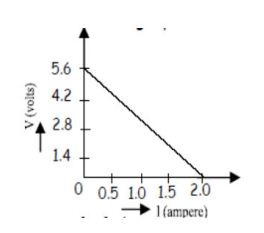i) 2.8 ohms

ii) 1.4 ohms

iii) 1.2 ohms

iv) zero

i) 2.8 ohms

Q 37. A 10 m long wire of uniform cross -section and 20 Ω resistance is used in a potentiometer. The wire is connected in series with a battery of 5 V along with an external resistance of 480 Ω. If an unknown emf E is balanced at 6.0 m length of the wire, then the value of unknown emf is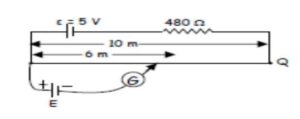i) 1.2 V

ii) 1.02 V

iii) 0.2 V

iv) 0.12 V

iv) 0.12 V

Q 38. The current sensitivity of a galvanometer increases by 20%. If its resistance also increases by 25%, the voltage sensitivity will

i) decrease by 1%

ii) increased by 5%

iii) increased by 10%

iv ) decrease by 4%

iv ) decrease by 4%

Q 39. Three infinitely long parallel straight current carrying wires A, B and C are kept at equal distance from each other as shown in the figure . The wire C experiences net force F .The net force on wire C, when the current in wire A is reversed will be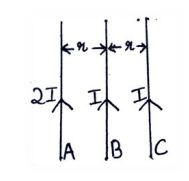i) Zero

ii) F/2

iii) F

iv) 2F

i) Zero

Q 40. In a hydrogen atom the electron moves in an orbit of radius 0.5 A0 making 10 revolutions per second, the magnetic moment associated with the orbital motion of the electron will be

i) 2.512 x 10-38 Am2

ii) 1.256 x 10-38 Am2

iii) 0.628 X10-38 Am2

iv) zero

ii) 1.256 x 10-38 Am2

Q 41. An air-cored solenoid with length 30 cm, area of cross-section 25 cm2 and number of turns 800, carries a current of 2.5 A. The current is suddenly switched off in a brief time of 10-3s. Ignoring the variation in magnetic field near the ends of the solenoid, the average back emf induced across the ends of the open switch in the circuit would be

i) zero

ii)3.125 volts

iii) 6.54 volts

iv) 16.74 volts

ii)3.125 volts

Q 42. A sinusoidal voltage of peak value 283 V and frequency 50 Hz is applied to a series LCR circuit in which R = 3 Ω, L = 25.48 mH, and C = 796 µF, then the power dissipated at the resonant condition will be-

i)39.70 kW

ii) 26.70 kW

iii)13.35 kW

iv)Zero

iii)13.35 kW

Q 43. A circular loop of radius 0.3cm lies parallel to much bigger circular of radius 20 cm. The centre of the small loop is on the axis of the bigger loop. The distance between their centres is 15 cm. If a current of 2.0 A flows through the smaller loop, then the flux linked with the bigger loop is

i) 3.3 X 10-11weber

ii) 6 X 10-11weber

iii) 6.6 X 10-9weber

iv) 9.1 X 10-11weber

iv) 9.1 X 10-11weber

Q 44. If both the number of turns and core length of an inductor is doubled keeping other factorsconstant, then its self-inductance will be-

i) Unaffected

ii) doubled

iii) halved

ii) doubled

45. Given below are two statements labelled as Assertion (A) and Reason (R)
Assertion (A): To increase the range of an ammeter, we must connect a suitable high resistance in series to it.
Reason (R): The ammeter with increased range should have high resistance.
Select the most appropriate answer from the options given below:

i) Both A and R are true and R is the correct explanation of A

ii) Both A and R are true but R is not the correct explanation of A.

iii)A is true but R is false.

iv) A is false and R is also false.

iv) A is false and R is also false.

46. Given below are two statements labelled as Assertion (A) and Reason (R)
Assertion (A): An electron has a high potential energy when it is at a location associated with a more negative value of potential, and a low potential energy when at a location associated with a more positive potential.
Reason (R):Electrons move from a region of higher potential to region of lower potential.

Select the most appropriate answer from the options given below:

i) Both A and R are true and R is the correct explanation of A

ii) Both A and R are true but R is not the correct explanation of A.

iii)A is true but R is false.

iv) A is false and R is also false.

iii)A is true but R is false.

47. Given below are two statements labelled as Assertion (A) and Reason (R)
Assertion(A): A magnetic needle free to rotate in a vertical plane, orients itself (with its axis) vertical at the poles of the earth.
Reason (R): At the poles of the earth the horizontal component of earth’s magnetic field will be zero.

Select the most appropriate answer from the options given below:

i) Both A and R are true and R is the correct explanation of A

ii) Both A and R are true but R is not the correct explanation of A.

iii)A is true but R is false.

iv) A is false and R is also false.

i) Both A and R are true and R is the correct explanation of A

48. Given below are two statements labelled as Assertion (A) and Reason (R)
Assertion(A): A proton and an electron, with same momenta, enter in a magnetic field in a direction at right angles to the lines of the force. The radius of the paths followed by them will be same.
Reason(R): Electron has less mass than the proton.

Select the most appropriate answer from the options given below:

i) Both A and R are true and R is the correct explanation of A

ii) Both A and R are true but R is not the correct explanation of A.

iii)A is true but R is false.

iv) A is false and R is also false.

ii) Both A and R are true but R is not the correct explanation of A.

49. Given below are two statements labelled as Assertion (A) and Reason (R)
Assertion (A):On Increasing the current sensitivity of a galvanometer by increasing the number of turns, may not necessarily increase its voltage sensitivity.
Reason(R ): The resistance of the coil of the galvanometer increases on increasing the number of turns.

Select the most appropriate answer from the options given below:

i) Both A and R are true and R is the correct explanation of A

ii) Both A and R are true but R is not the correct explanation of A.

iii)A is true but R is false.

iv) A is false and R is also false.

i) Both A and R are true and R is the correct explanation of A

SECTION C

This section consists of 6 multiple choice questions with an overall choice to attempt any 5. In case more than desirable number of questions are attempted, ONLY first 5 will be considered for evaluation.

Q 50. A small object with charge q and weight mg is attached to one end of a string of length ‘L’ attached to a stationary support. The system is placed in a uniform horizontal electric field ‘E’, as shown in the accompanying figure. In the presence of the field, the string makes a constant angle θ with the vertical. The sign and magnitude of q-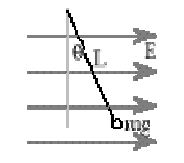i) positive with magnitude mg/E

ii) positive with magnitude (mg/E)tanθ

iii) negative with magnitude mg/E tanθ

iv) positive with magnitude E tanθ/mg

ii) positive with magnitude (mg/E)tanθ

Q 51. A free electron and a free proton are placed between two oppositely charged parallel plates. Both are closer to the positive plate than the negative plate.Which of the following statements is true?
I. The force on the proton is greater than the force on the electron.
II. The potential energy of the proton is greater than that of the electron.
III. The potential energy of the proton and the electron is the same.

i) I only

ii) II only

iii) III and I only

iv)II and I only

ii) II only

Case study :The large-scale transmission and distribution of electrical energy over long distances is done with the use of transformers. The voltage output of the generator is stepped-up. It is then transmitted over long distances to an area sub-station near the consumers. There the voltage is stepped down. It is further stepped down at distributing sub-stations and utility poles before a power supply of 240 V reaches our homes.

Q 52. Which of the following statement is true?

i) Energy is created when a transformer steps up the voltage

ii) A transformer is designed to convert an AC voltage to DC voltage

iii) Step–up transformer increases the power for transmission

iv) Step–down transformer decreases the AC voltage

iv) Step–down transformer decreases the AC voltage

Q 53. If the secondary coil has a greater number of turns than the primary,

i) the voltage is stepped-up (Vs >Vp ) and arrangement is called a step-up transformer

ii) the voltage is stepped-down (Vs <Vp ) and arrangement is called a step- down transformer

iii) the current is stepped-up (Is >Ip ) and arrangement is called a step-up transformer

iv) the current is stepped-down (Is <Ip ) and arrangement is called a step- down transformer

i) the voltage is stepped-up (Vs >Vp ) and arrangement is called a step-up transformer

Q 54. We need to step-up the voltage for power transmission, so that

i) the current is reduced and consequently, the I 2 R loss is cut down

ii) the voltage is increased , the power losses are also increased

iii) the power is increased before transmission is done

iv) the voltage is decreased so V2 /R losses are reduced

i) the current is reduced and consequently, the I 2 R loss is cut down

Q 55. A power transmission line feeds input power at 2300 V to a step down transformer with its primary windings having 4000 turns. The number of turns in the secondary in order to get output power at 230 V are

i) 4

ii) 40

iii) 400

iv) 4000

1.suraj kumar says: